# Calculus Without Tears

### The stress and strain tensors

#### 1-D stress and strain

When a force is applied to the end of a 1-D elastic object, i.e. a spring, anchored at the other end, the points in the spring move (are displaced), and at each point in the spring there is tension (stress) and distortion (strain).

The strain on the stretched or compressed spring can be defined as the change in length when the spring is stresed, i.e. stretched or compressed, divided by the original length of the spring when it is unstressed.

That's a global definition. We want a local definition that defines the strain on the spring at each point in the spring. To that end we define the displacement function for each point in the spring, for a point p with coordinate x, D(x) is defined as the coordinate of p in the stressed spring minux x. That is, D(x) is the distance the point p at location x is displaced in the stressed spring.

For example, if a spring of length L is stretched to a length of L + e, then the displacement function appears as follows: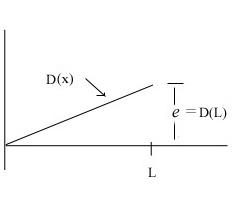The global stress on the spring is e / L, and if we define the stress at a point p in the spring with coordinate x as the derivative of the displacement function at x, that is as dD(x)/dx, then the locally defined stress at each point will equal the globally defined stress and we have an local definition of stress that matches our intuition.

The global stress on the spring equals the force applied to the spring, and this force is replicated at each point in the spring as the tension at that point.

Things are very simple with springs.

#### 2-D stress and strain

The 1-D case suggests defining strain in the 2-D case with the partial derivatives of the displacement function. The displacement function for a 2-D stressed object is also 2-D, that is, a vector, and can be written as
D(x,y) = (u(x,y), v(x,y))
Note: this is the standard notation.

The partial derivatives of a vector function arranged in a 2x2 matrix make up the Jacobian, which for the displacement function D(x,y) = (u(x,y), v(x,y)) is given by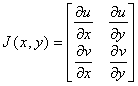The Jacobian J(x,y) of D maps a unit vector r = (rx,ry) to the directional derivative of D in the r direction.The directional derivative of the displacement function is a vector function. Interpreted geometrically, the component of J(x,y)*r parallel to r represents the stretching/compressing component of the strain at (x,y), and the component perpendicular to r represents the twisting strain.

The first thing to note is that we are representing a physical property of the stressed object, the strain at a point, by a 2x2 matrix. That is, a number won't suffice, a vector won't suffice, and a 2x2 matrix is required to represent the strain state of an object at a point. You're familiar with representing physical properties with numbers and vectors, but probably not with representing physical properties with 2x2 matrices, so, this is something new.

It's not really the matrix that represents the property, it is the map from unit vectors to directional derivatives, and this map is defined by the matrix for the given coordinate system. If we change coordinates, the components of the matrix will change, but the map from unit vectors to directional derivatives will not change. You are likely familiar the converting vector components when there is a changes of coordinates, and the conversion of matrix components is similar and given in Appendix III. When matrices, interpreted as vector maps, are used to represent physical quantities they are called tensors. Thus we have tentively defined the strain tensor at a point in a stressed object as the Jacobian of the displacment function D(x,y) = (u(x,y), v(x,y)).

However, there is a little problem with this definition that requires a change. Notice the following - if a force is applied to an object through a traction, and the object moves vertically or horizontally but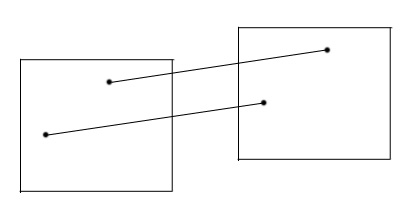is otherwise unchanged, the displacement vector is the same for every point in the object, hence the Jacobian is 0 at every point, corresponding to 0 strain at every point and all is well.

However, suppose the box rotates and is otherwise unchanged. The displacement vector is not constant for a rotation, hence its derivatives are not 0 and the Jacobian is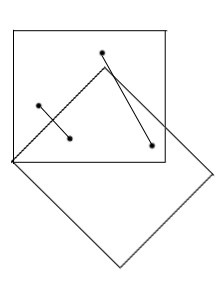non-zero for every point in the object. However there is no strain on the rotated object. So, the Jacobian, which is non-zero, is not an ideal candidate for the strain tensor, which must be 0 if the object is not stressed.

The solution is to partition the Jacobian into a symmetric strain tensor and an anti-symmetric rotation tensor –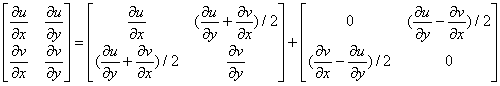∂u/ ∂x is the normal strain in the x direction.

∂v / ∂y is the normal strain in the y direction.

The shear strain θ is defined as the change in angle between the x and y axes in the stressed object. In the diagram the shear strain is, using the small angle approximation, θ = θx + θy = ∂u/∂y + ∂v/∂x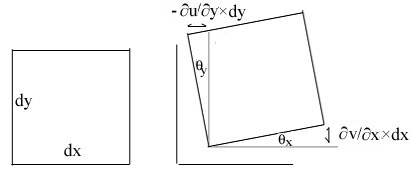Note that when the object is rotated θy = -θy and so ∂u/∂y + ∂v/∂x = 0, and the shear strain is correctly computed as 0.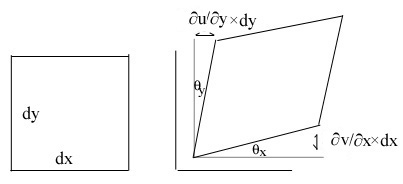Thus the strain tensor is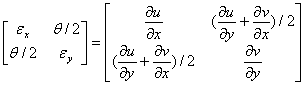When force is applied to a 3-D (2-D) object it can't be applied at a point, it is applied over an area (line) and is called a traction and has units F/m2 (F/m).

The internal stress at a point in a stressed object is inferred from the strain on the object at that point. In 2-D the strain at a point is direction dependent, and hence the stress causing the strain must be direction dependent, and is represented by a tensor. In 2-D the stress tensor maps a unit vector to a vector having units F/m representing the stress in that direction. The relationship between stress and strain is given by Hooke's Law. Hooke's Law is fully expressed in 3-D, the 2-D form used in the book is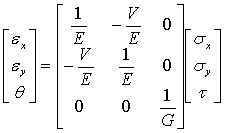where E = Young’s modulus
V = Poisson’s ratio
G = shear modulus
εx, εy, σx, σy are normal strains and stresses
θ, τ are shear strain and stress